GeometrizeTwitterBot  1.0 Python Twitter bot for geometrizing images into geometric primitives
geometrize Namespace Reference

Module that interfaces with the Geometrize executable, composing and executing scripts on it to geometrize images. More...

Functions

def _run_geometrize_code (code)
Executes the given script source code. More...

def _compose_image_path (filename)

Loads up an image from the given filepath. More...

def run_geometrize (code, tags=None)
Executes the given script source code. More...

def test_geometrize ()
Performs some basic tests to ensure that Geometrize can run scripts and turn images into shapes properly. More...

Detailed Description

Module that interfaces with the Geometrize executable, composing and executing scripts on it to geometrize images.

◆ _compose_image_path()

 def geometrize._compose_image_path ( filename )
private
Here is the call graph for this function: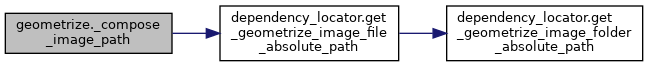Here is the caller graph for this function: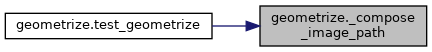private

Loads up an image from the given filepath.

:return The loaded image, or None if the image failed to load.

25  image = None
27  try:
28  image = Image.open(filepath)
29  except:
31  return image
32
Here is the caller graph for this function: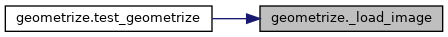◆ _run_geometrize_code()

 def geometrize._run_geometrize_code ( code )
private

Executes the given script source code.

:return The Geometrize process return code, 0 indicates success.

14 def _run_geometrize_code(code):
16  script_inline_flag = "--script_inline"
17  return call([executable_path, script_inline_flag, code])
18
Here is the call graph for this function: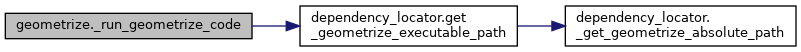Here is the caller graph for this function: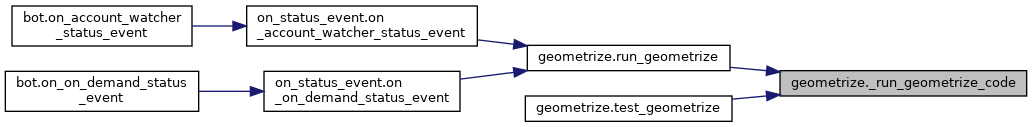◆ run_geometrize()

 def geometrize.run_geometrize ( code, tags = None )

Executes the given script source code.

:return True if the script executed successfully, else false.

35 def run_geometrize(code, tags = None):
36  if tags is not None:
37  code = script_wrangler.replace_tags(code, tags)
38
40  print("Script has template tags that have not been replaced, will fail rather than run script")
41  return False
42
43  print("Will run script")
44  print(code)
45  ret_code = _run_geometrize_code(code)
46
47  if ret_code != 0:
48  print("Failed to execute test script")
49  return False
50
51  return True
52
Here is the call graph for this function: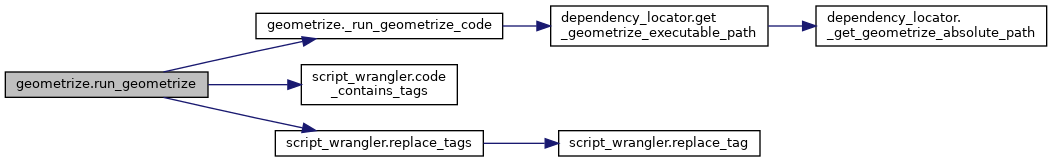Here is the caller graph for this function: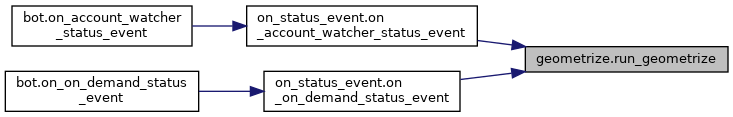◆ test_geometrize()

 def geometrize.test_geometrize ( )

Performs some basic tests to ensure that Geometrize can run scripts and turn images into shapes properly.

:return True if the tests executed successfully, else false.

55 def test_geometrize():
58  if not code:
59  print("Failed to read test script, test will fail")
60  return False
61
62  image_input_path = _compose_image_path("test_image_in.png")
63  image_output_path = _compose_image_path("test_image_out.png")
64
67  if input_image is None:
68  print("Failed to read the input image, test script will fail")
69  return False
70
71  print("Replacing script tags")
72
73  # Note that the backslashes in image paths need to be escaped, or just use forward slashes.
74  # This is because we are putting them into string literals in the scripts.
75  code = script_wrangler.replace_tag(code, "::IMAGE_INPUT_PATH::", image_input_path)
76  code = script_wrangler.replace_tag(code, "::IMAGE_OUTPUT_PATH::", image_output_path)
77
79  print("Failed to replace all template tags in script, script will fail")
80  return False
81
83  print("Failed to replace all template tags in script, script will fail")
84  return False
85
86  ret_code = _run_geometrize_code(code)
87
88  if ret_code != 0:
89  print("Failed to execute test script")
90  return False
91
92  print("Checking if the test script saved the result image correctly")
93
95  if output_image is None:
96  print("Failed to read the resulting geometrized image, test script will fail")
97  return False
98
99  print("Printing input image to console\r\n")
101
102  print("Printing Geometrized image to console\r\n")
104
105  return True
Here is the call graph for this function: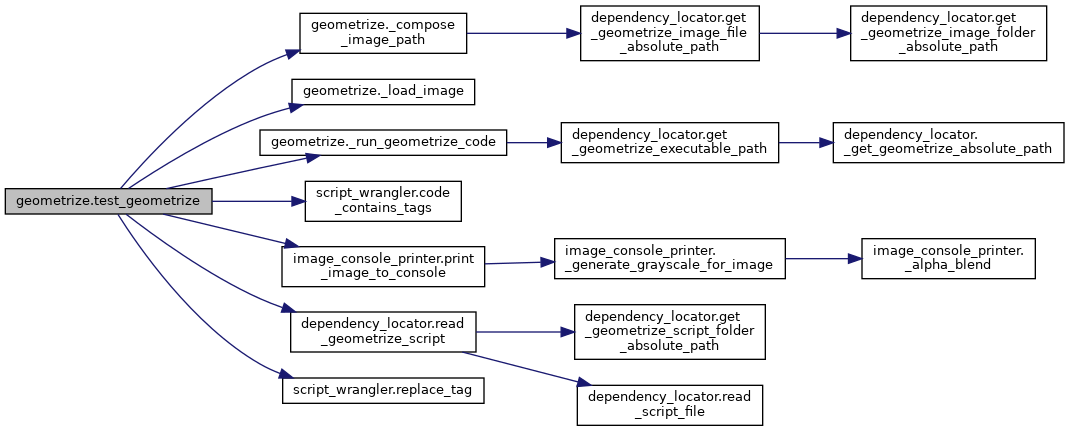dependency_locator.get_geometrize_executable_path
def get_geometrize_executable_path()
Gets the absolute path to where we expect to find the Geometrize executable.
Definition: dependency_locator.py:28
geometrize.test_geometrize
def test_geometrize()
Performs some basic tests to ensure that Geometrize can run scripts and turn images into shapes prope...
Definition: geometrize.py:55
image_console_printer.print_image_to_console
def print_image_to_console(img)
Definition: image_console_printer.py:82
geometrize._compose_image_path
def _compose_image_path(filename)
Definition: geometrize.py:19
geometrize.run_geometrize
def run_geometrize(code, tags=None)
Executes the given script source code.
Definition: geometrize.py:35
script_wrangler.replace_tag
def replace_tag(code, tag, value)
Replaces all instances of a template ::TAG:: in the given code with the given value.
Definition: script_wrangler.py:8
dependency_locator.get_geometrize_image_file_absolute_path
def get_geometrize_image_file_absolute_path(filename)
Composes an absolute path for an image file in the image data folder.
Definition: dependency_locator.py:42
script_wrangler.code_contains_tags
def code_contains_tags(code)
Returns true if the given code contains any ::TAG::s, else false.
Definition: script_wrangler.py:27
Loads up an image from the given filepath.
Definition: geometrize.py:24
geometrize._run_geometrize_code
def _run_geometrize_code(code)
Executes the given script source code.
Definition: geometrize.py:14
script_wrangler.replace_tags
def replace_tags(code, dict)
Replaces all instances of template ::TAG::s in the code from the given dictionary with their correspo...
Definition: script_wrangler.py:12